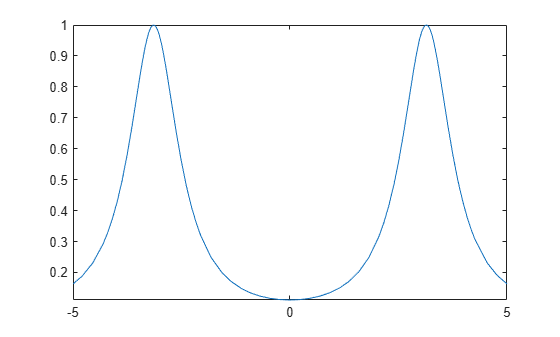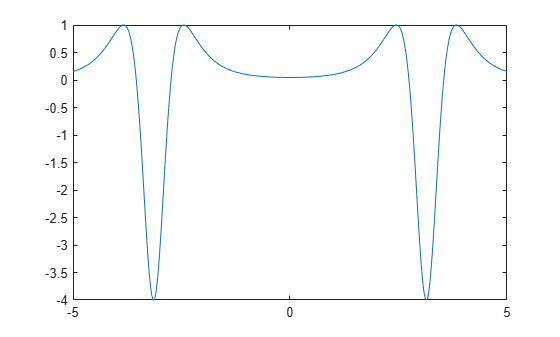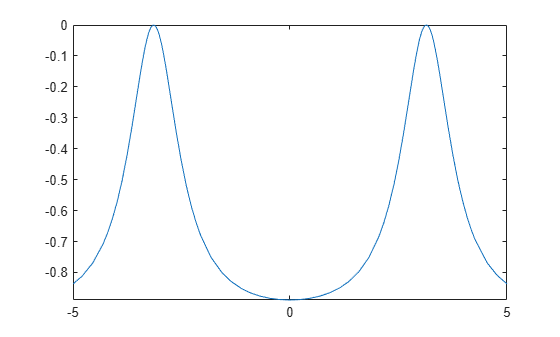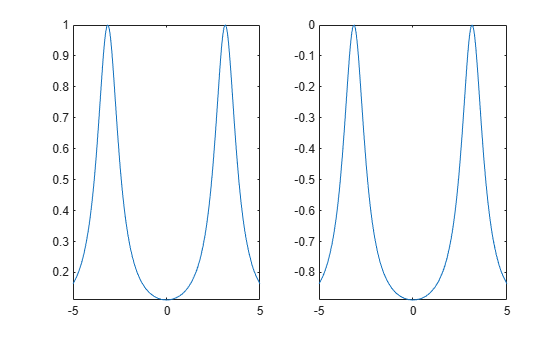Documentation

Learn Calculus in the Live Editor

Learn calculus and applied mathematics using the Symbolic Math Toolbox™. The example shows introductory functions fplot and diff.

To manipulate a symbolic variable, create an object of type syms.

syms x

Once a symbolic variable is defined, you can build and visualize functions with fplot.

f(x) = 1/(5+4*cos(x))
f(x) =

$\frac{1}{4 \mathrm{cos}\left(x\right)+5}$

fplot(f)Evaluate the function at $x=\pi /2$ using math notation.

f(pi/2)
ans =

$\frac{1}{5}$

Many functions can work with symbolic variables. For example, diff differentiates a function.

f1 = diff(f)
f1(x) =

$\frac{4 \mathrm{sin}\left(x\right)}{{\left(4 \mathrm{cos}\left(x\right)+5\right)}^{2}}$

fplot(f1)diff can also find the ${N}^{th}$ derivative. Here is the second derivative.

f2 = diff(f,2)
f2(x) =

$\frac{4 \mathrm{cos}\left(x\right)}{{\left(4 \mathrm{cos}\left(x\right)+5\right)}^{2}}+\frac{32 {\mathrm{sin}\left(x\right)}^{2}}{{\left(4 \mathrm{cos}\left(x\right)+5\right)}^{3}}$

fplot(f2)int integrates functions of symbolic variables. The following is an attempt to retrieve the original function by integrating the second derivative twice.

g = int(int(f2))
g(x) =

$-\frac{8}{{\mathrm{tan}\left(\frac{x}{2}\right)}^{2}+9}$

fplot(g)At first glance, the plots for $f$ and $g$ look the same. Look carefully, however, at their formulas and their ranges on the y-axis.

subplot(1,2,1)
fplot(f)
subplot(1,2,2)
fplot(g)$e$ is the difference between $f$ and $g$. It has a complicated formula, but its graph looks like a constant.

e = f - g
e(x) =

$\frac{8}{{\mathrm{tan}\left(\frac{x}{2}\right)}^{2}+9}+\frac{1}{4 \mathrm{cos}\left(x\right)+5}$

To show that the difference really is a constant, simplify the equation. This confirms that the difference between them really is a constant.

e = simplify(e)
e(x) = $1$

Mathematical Modeling with Symbolic Math Toolbox

Get examples and videos